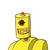# The ratio of the ages of Suresh and his fa-ther is 2:7. The difference between theirages is 40 years. Then, the ratio

The ratio of the ages of Suresh and his fa-
ther is 2:7. The difference between their
ages is 40 years. Then, the ratio of their
ages 6 years ago was?
1) 2:3 2) 2:1 3)1:3 4 1:5
2​

### 1 thought on “The ratio of the ages of Suresh and his fa-<br />ther is 2:7. The difference between their<br />ages is 40 years. Then, the ratio”

1.### Given:–

Ratio of ages of Suresh and his father=2:7

Difference=40 years

### Assumption:–

Let the age of Suresh be 2x and his father be 7x

### Accordingtoquestion:–

7x-2x=40

5x=40

$$x = \frac{40}{5}$$

x=8

Age of Suresh=2x

=2(8)

=16 years

Age of his father=7x

=7(8)

=56 years

### Therefore:–

Age of Suresh before 6 years=16-6

=10 years

Age of his father before 6 years=56-6

=50 years

= 1:5